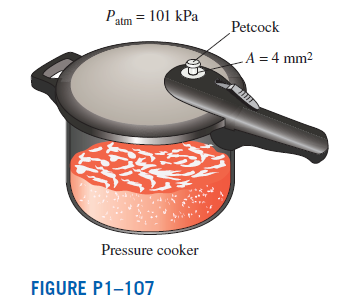×
Get Full Access to Thermodynamics: An Engineering Approach - 8 Edition - Chapter 1 - Problem 107
Get Full Access to Thermodynamics: An Engineering Approach - 8 Edition - Chapter 1 - Problem 107

×

# Solved: A pressure cooker cooks a lot faster than anISBN: 9780073398174 56

## Solution for problem 107 Chapter 1

Thermodynamics: An Engineering Approach | 8th Edition

• Textbook Solutions
• 2901 Step-by-step solutions solved by professors and subject experts
• Get 24/7 help from StudySoup virtual teaching assistantsThermodynamics: An Engineering Approach | 8th Edition

4 5 1 290 Reviews
29
1
Problem 107

A pressure cooker cooks a lot faster than an ordinary pan by maintaining a higher pressure and temperature inside. The lid of a pressure cooker is well sealed, and steam can escape only through an opening in the middle of the lid. A separate metal piece, the petcock, sits on top of this opening and prevents steam from escaping until the pressure force overcomes the weight of the petcock. The periodic escape of the steam in this manner prevents any potentially dangerous pressure buildup and keeps the pressure inside at a constant value. Determine the mass of the petcock of a pressure cooker whose operation pressure is $$100 \mathrm{kPa}$$ gage and has an opening cross-sectional area of $$4 \mathrm{~mm}^{2}$$. Assume an atmospheric pressure of $$101 \mathrm{kPa}$$, and draw the free-body diagram of the petcock.Equation Transcription:Text Transcription:

100 kPa

4 mm^2

101 kPa

Step-by-Step Solution:
Step 1 of 3

Physics 2 for Engineering Week 7 Ɛ – V r V = R Ɛ – Ir – IR = 0 V abƐ – Ir (V is the terminal voltage) ab Power = Energy / Time = qV/t = IV Power supplied by battery P batt =I 2 2 Power supplied by battery due to internal resistance r, P = V Ir= I rr= V /r r 2 2 Power dissipated in resistor R, P = VRI = IRR = V /R R

Step 2 of 3

Step 3 of 3

##### ISBN: 9780073398174

The answer to “?A pressure cooker cooks a lot faster than an ordinary pan by maintaining a higher pressure and temperature inside. The lid of a pressure cooker is well sealed, and steam can escape only through an opening in the middle of the lid. A separate metal piece, the petcock, sits on top of this opening and prevents steam from escaping until the pressure force overcomes the weight of the petcock. The periodic escape of the steam in this manner prevents any potentially dangerous pressure buildup and keeps the pressure inside at a constant value. Determine the mass of the petcock of a pressure cooker whose operation pressure is $$100 \mathrm{kPa}$$ gage and has an opening cross-sectional area of $$4 \mathrm{~mm}^{2}$$. Assume an atmospheric pressure of $$101 \mathrm{kPa}$$, and draw the free-body diagram of the petcock. Equation Transcription:Text Transcription:100 kPa4 mm^2101 kPa” is broken down into a number of easy to follow steps, and 140 words. The full step-by-step solution to problem: 107 from chapter: 1 was answered by , our top Engineering and Tech solution expert on 08/01/17, 09:10AM. This full solution covers the following key subjects: pressure, petcock, steam, cooker, opening. This expansive textbook survival guide covers 17 chapters, and 2295 solutions. This textbook survival guide was created for the textbook: Thermodynamics: An Engineering Approach , edition: 8. Thermodynamics: An Engineering Approach was written by and is associated to the ISBN: 9780073398174. Since the solution to 107 from 1 chapter was answered, more than 704 students have viewed the full step-by-step answer.

## Discover and learn what students are asking

Calculus: Early Transcendental Functions : Integration by Tables and Other Integration Techniques
?In Exercises 47-52, verify the integration formula. \(\int \frac{1}{\left(u^{2} \pm a^{2}\right)^{3 / 2}} d u=\frac{\pm u}{a^{2} \sqrt{u^{2}

Statistics: Informed Decisions Using Data : Properties of the Normal Distribution
?In Problems 25–28, the graph of a normal curve is given. Use the graph to identify the values of ? and ?.

Unlock Textbook Solution

Enter your email below to unlock your verified solution to:

Solved: A pressure cooker cooks a lot faster than an